##### Statistics: 1001 Practice Problems For Dummies (+ Free Online Practice)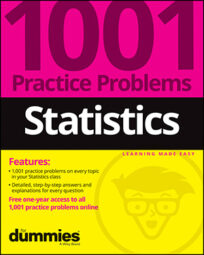Solving statistics problems can involve finding probabilities, mean, and standard deviation for a specific random variable, in this case the binomial. Solve the following problems about the mean, standard deviation, and variance of binomial random variables.

## Sample questions

1. What is the mean of a binomial random variable with n = 18 and p = 0.4?

The mean of a binomial random variable X is represented by the symbol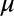A binomial distribution has a special formula for the mean, which is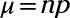Here, n = 18 and p = 0.4, so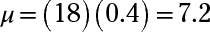2. What is the standard deviation of a binomial distribution with n = 18 and p = 0.4? Round your answer to two decimal places.

The standard deviation of X is represented by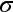and represents the square root of the variance. If X has a binomial distribution, the formula for the standard deviation is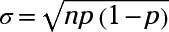where n is the number of trials and p is the probability of success on each trial. For this situation, n = 18 and p = 0.4, so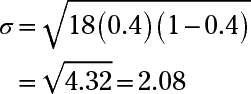3. What is the variance of a binomial distribution with n = 25 and p = 0.35? Round your answer to two decimal places.

The variance is represented by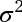and represents the typical squared distance from the mean for all values of X.

For a binomial distribution, the variance has its own formula: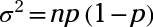In this case, n = 25 and p = 0.35, so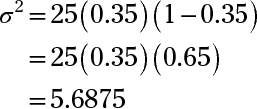Rounded to two decimal places, the answer is 5.69.

4. A binomial distribution with p = 0.14 has a mean of 18.2. What is n?

The mean of a random variable X is denotedFor a binomial distribution, the mean has a special formula:In this case, p = 0.14 andis 18.2, so you need to find n. Plug the known values into the formula for the mean, so 18.2 = n(0.14), and then divide both sides by 0.14 to get n = 18.2/0.14 = 130.

If you need more practice on this and other topics from your statistics course, visit 1,001 Statistics Practice Problems For Dummies to purchase online access to 1,001 statistics practice problems! We can help you track your performance, see where you need to study, and create customized problem sets to master your stats skills.